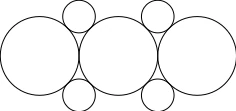# [原创]在 TikZ 中利用 scope 环境实现平移做图TikZ 宏包除了提供 tikzpicture 环境外还提供了一个可以嵌套在 tikzpicture 里的子环境 scope, 简单点说就是可以通过 scope 环境把同一标准的画图命令放在一起, 而只需要声明一次标准, 比如 scale, thick, -> 等等之类. 个人用的更多的是通过 scope 环境完成平移做图, 当然命令不多的的时候也可以直接平移做图, 如上图中圆心位于点 $(1,1)$ 的圆.

TikZ 宏包还提供了一个 scopes library, 以简化 scope 环境的调用, 当个人觉得直接显示 scope 似乎更有利于代码的可读性.

\documentclass{standalone}

\usepackage{tikz}

\begin{document}

\begin{tikzpicture}
\draw (0,0) circle (1cm);
\draw [shift={(1,1)}] (0,0) circle (0.414cm);
\begin{scope}[xshift=-1cm,yshift=1cm]
\draw (0,0) circle (0.414cm);
\end{scope}
\begin{scope}[shift=(225:{sqrt(2)})]
\draw (0,0) circle (0.414cm);
\end{scope}
\begin{scope}[shift={(1,-1)}]
\draw (0,0) circle (0.414cm);
\end{scope}

\begin{scope}[xshift=2cm]
\draw (0,0) circle (1cm);
\end{scope}
\begin{scope}[xshift=-2cm]
\draw (0,0) circle (1cm);
\end{scope}
\end{tikzpicture}

\end{document}

\coordinate (A) at (3,4);

\documentclass{standalone}

\usepackage{tikz}

\begin{document}

\begin{tikzpicture}
\draw (0,0) circle (1);
\draw [-stealth] (-1,-1) -- ++(2,2);
\node [below] at (0,-1) {(a)};
\begin{scope}[xshift=3cm]
\draw (0,0) circle (1);
\draw [-stealth] (-1,-1) -- ++(2,2);
\node [below] at (0,-1) {(b)};
\end{scope}
\end{tikzpicture}

\end{document}